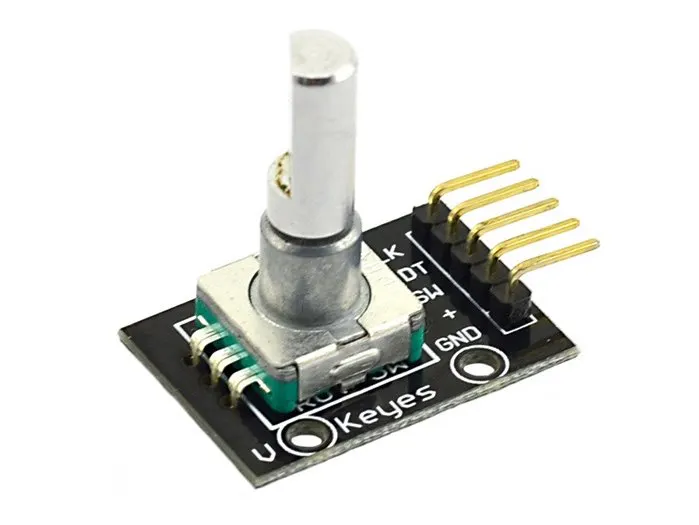# How to Use a Rotary Encoder

In this little project, I'll show how to use a rotary encoder.

• 4,828 views
• 2 comments
• 11 respects

## About this project

A rotary encoder has a number defined positions for a complete revolution (a turn of 360 degrees). You can feel a click at each step when turning the cursor of the potentiometer.

Here we don't measure the value of a variable resistance, we measure logical signals on two digital pins of the Arduino.

For example, when one is in a position, A and B have the same logical level ( 0 or 1 ).

If you turn clockwise, the status signal A changes before B, and conversely when we turn in the other direction.

In this project, we'll display the angular positions on the serial monitor.

### Connections

Rotary encoder --> Arduino

CLK --> Digital 4

DT --> Digital 5

SW --> Digital 0

+ --> 5V

GND --> GND

## Code

##### Rotary encoderArduino
```int val;
int PinCLK = 4;
int PinDT = 5;
int PinSW = 0;
static long encoderPos = -1;    // Au 1er démarrage, il passera à 0
int PinCLKLast = LOW;
int nbPas = 20;                 // Résolution de l'encodeur
int n = LOW;

void setup() {
pinMode (PinCLK,INPUT);
pinMode (PinDT,INPUT);
pinMode (PinSW,INPUT);
Serial.begin (9600);
}

void loop() {
if (!(digitalRead(PinSW))) {
encoderPos = 0;

}

n = digitalRead(PinCLK);

if ((PinCLKLast == LOW) && (n == HIGH)) {

if (digitalRead(PinDT) == LOW) {
encoderPos--;
if ( encoderPos < 0 ) {
encoderPos = nbPas;
}
} else {
encoderPos++;
if ( encoderPos > ( nbPas - 1 ) ) {
encoderPos = 0;
}
}
Serial.print (encoderPos);
Serial.print(", angle ");
Serial.println( abs ( encoderPos * ( 360 / nbPas ) ) );

}
PinCLKLast = n;
}
```

## Comments

by Andrewf1

• 11,652 views
• 1 comment
• 25 respects

• 15,543 views
• 2 comments
• 35 respects

#### DIY Simple Measuring Wheel with Rotary Encoder

Project tutorial by Mirko Pavleski

• 17,049 views
• 6 comments
• 22 respects

• 7,493 views
• 2 comments
• 18 respects

• 5,937 views
• 0 comments
• 15 respects

#### Arduino Menu on a Nokia 5110 Lcd Using a Rotary Encoder

Project tutorial by Nick Koumaris

• 24,682 views
• 10 comments
• 56 respects